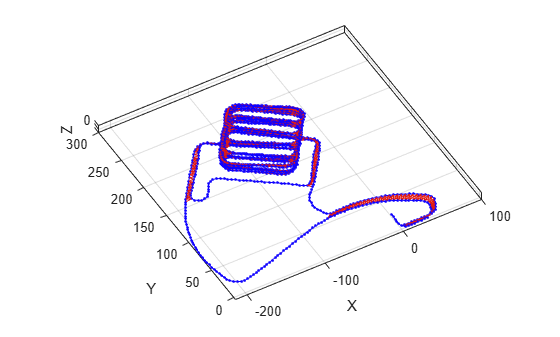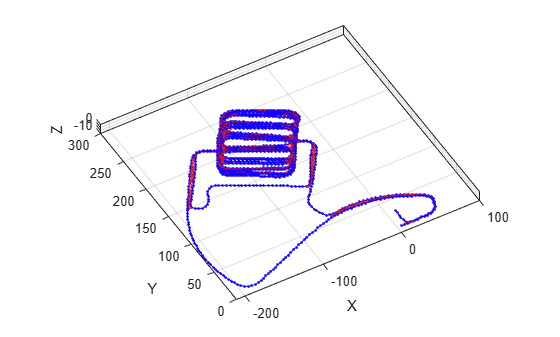# poseGraph3D

Create 3-D pose graph

## Description

A `poseGraph3D` object stores information for a 3-D pose graph representation. A pose graph contains `nodes` connected by `edges`, with edge constraints that define the relative pose between nodes and the uncertainty on that measurement. The `optimizePoseGraph` function modifies the nodes to account for the uncertainty and improve the overall graph.

For 2-D pose graphs, see `poseGraph`.

To construct a pose graph iteratively, use `addRelativePose` to add poses and connect them to the existing graph. Specify the uncertainty associated using an information matrix. Specify loop closures by add extra edge constraints between existing nodes.

## Creation

### Syntax

``poseGraph = poseGraph3D``
``poseGraph = poseGraph3D('MaxNumEdges',maxEdges,'MaxNumNodes',maxNodes)``

### Description

example

``` `poseGraph = poseGraph3D` creates a 3-D pose graph object. Add poses using `addRelativePose` to construct a pose graph iteratively.```
``` `poseGraph = poseGraph3D('MaxNumEdges',maxEdges,'MaxNumNodes',maxNodes)` specifies an upper bound on the number of edges and nodes allowed in the pose graph when generating code. This limit is only required when generating code.```

## Properties

expand all

Number of nodes in pose graph, specified as a positive integer. Each node represents a pose in the pose graph as an ```[x y z qw qx qy qz]``` vector with an xyz-position and quaternion orientation, `[qw qx qy qz]`. To specify relative poses between nodes, use `addRelativePose`. To get a list of all nodes, use `nodes`.

Note

The order of the quaternion `[qw qx qy qz]` uses the standard convention. Some vehicle coordinate systems instead specify the order as `[qx qy qz qw]`. Check the source of your pose graph data before adding nodes to your `poseGraph3D` object.

Number of edges in pose graph, specified as a nonnegative integer. Each edge connects two nodes in the pose graph. Loop closure edges are included.

Number of loop closures in pose graph, specified as a nonnegative integer. To get the edge IDs of the loop closures, use the `LoopClosureEdgeIDs` property.

Loop closure edges IDs, specified as a vector of edge IDs.

## Object Functions

 `addRelativePose` Add relative pose to pose graph `edges` Edges in pose graph `edgeConstraints` Edge constraints in pose graph `edgeResidualErrors` Compute pose graph edge residual errors `findEdgeID` Find edge ID of edge `nodes` Poses of nodes in pose graph `optimizePoseGraph` Optimize nodes in pose graph `removeEdges` Remove loop closure edges from graph `show` Plot pose graph

## Examples

collapse all

Optimize a pose graph based on the nodes and edge constraints. The pose graph used in this example is taken from the MIT Dataset and was generated using information extracted from a parking garage.

Load the pose graph from the MIT dataset. Inspect the `poseGraph3D` object to view the number of nodes and loop closures.

```load parking-garage-posegraph.mat pg disp(pg);```
``` poseGraph3D with properties: NumNodes: 1661 NumEdges: 6275 NumLoopClosureEdges: 4615 LoopClosureEdgeIDs: [1x4615 double] ```

Plot the pose graph with IDs off. Red lines indicate loop closures identified in the dataset.

```title('Original Pose Graph') show(pg,'IDs','off'); view(-30,45)```Optimize the pose graph. Nodes are adjusted based on the edge constraints and loop closures. Plot the optimized pose graph to see the adjustment of the nodes with loop closures.

```updatedPG = optimizePoseGraph(pg); figure title('Updated Pose Graph') show(updatedPG,'IDs','off'); view(-30,45)```Carlone, Luca, Roberto Tron, Kostas Daniilidis, and Frank Dellaert. "Initialization Techniques for 3D SLAM: a Survey on Rotation Estimation and its Use in Pose Graph Optimization." 2015 IEEE International Conference on Robotics and Automation (ICRA). 2015, pp. 4597–4604.